# RD Sharma Solutions for Class 11 Maths Chapter 22 Brief review of Cartesian System of Rectangular Coordinates

## RD Sharma Solutions Class 11 Maths Chapter 22 – Download Free PDF Updated for 2021-22

RD Sharma Solutions for Class 11 Maths Chapter 22 – Brief review of Cartesian System of Rectangular Coordinates are provided here for students to study and score good marks in the board exams. The RD Sharma solutions which contain a huge number of solved examples and illustrations provide stepwise explanations of various difficult concepts and comprises a wide variety of questions for practice.

This chapter primarily deals with problems using section formula and finding the area of a triangle. RD Sharma Solutions are completely based on the exam-oriented approach to help students acquire good marks in their board examination. The pdf of RD Sharma Class 11 Maths Solutions is provided here. Practising these problems will ensure that students can easily excel in their final exams. Students can refer and download this chapter pdf from the below-mentioned links.

Chapter 22 – Brief review of Cartesian System of Rectangular Coordinates contains three exercises and the RD Sharma Solutions present in this page provide solutions to the questions present in each exercise. Now, let us have a look at the concepts discussed in this chapter.

• Cartesian coordinate system.
• Distance between two points.
• Area of a triangle.
• Section formula.
• Certroid, in-centre and ex-centres of a triangle.
• Locus and equation to a locus.
• Shifting of origin.

## Download the pdf of RD Sharma Solutions for Class 11 Maths Chapter 22 – Brief review of Cartesian System of Rectangular Coordinates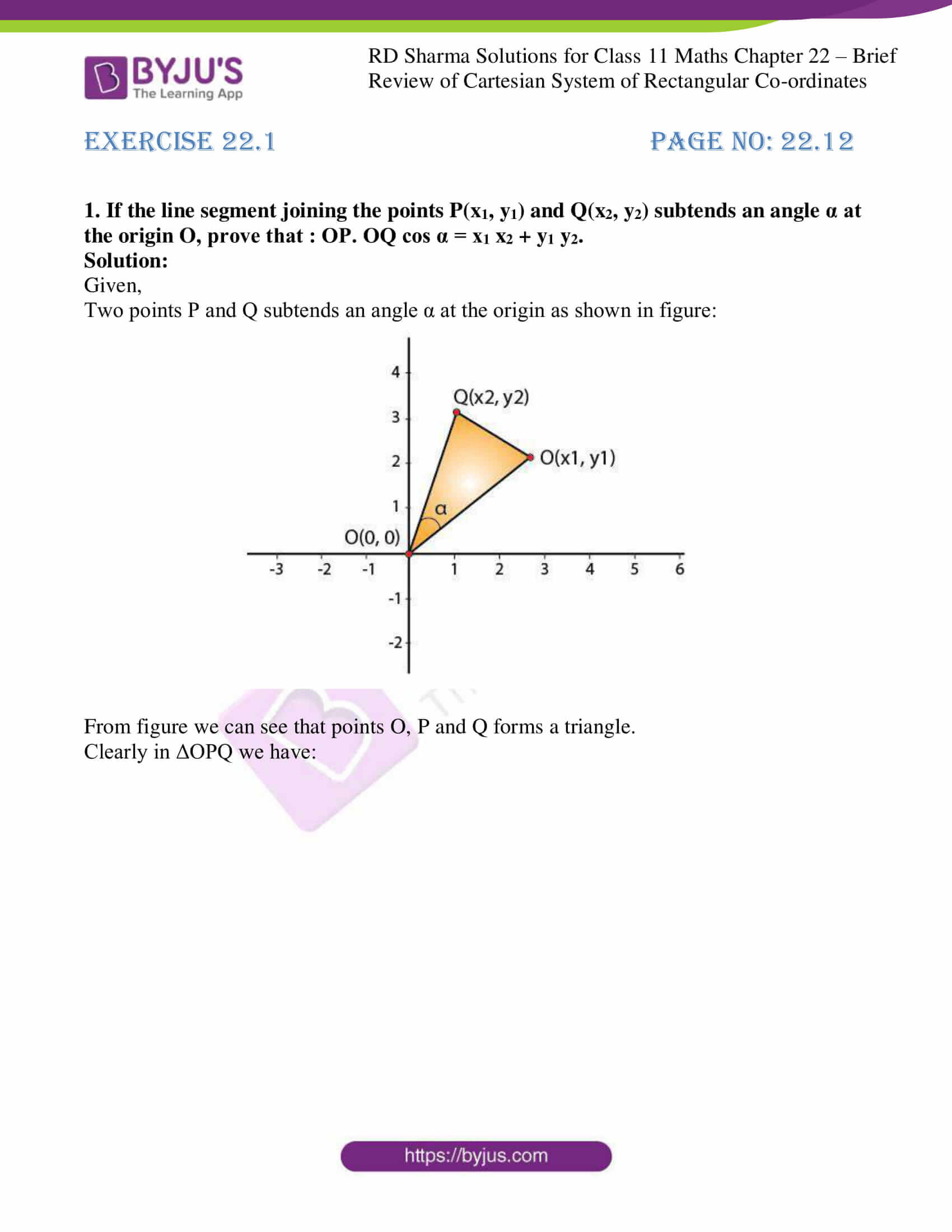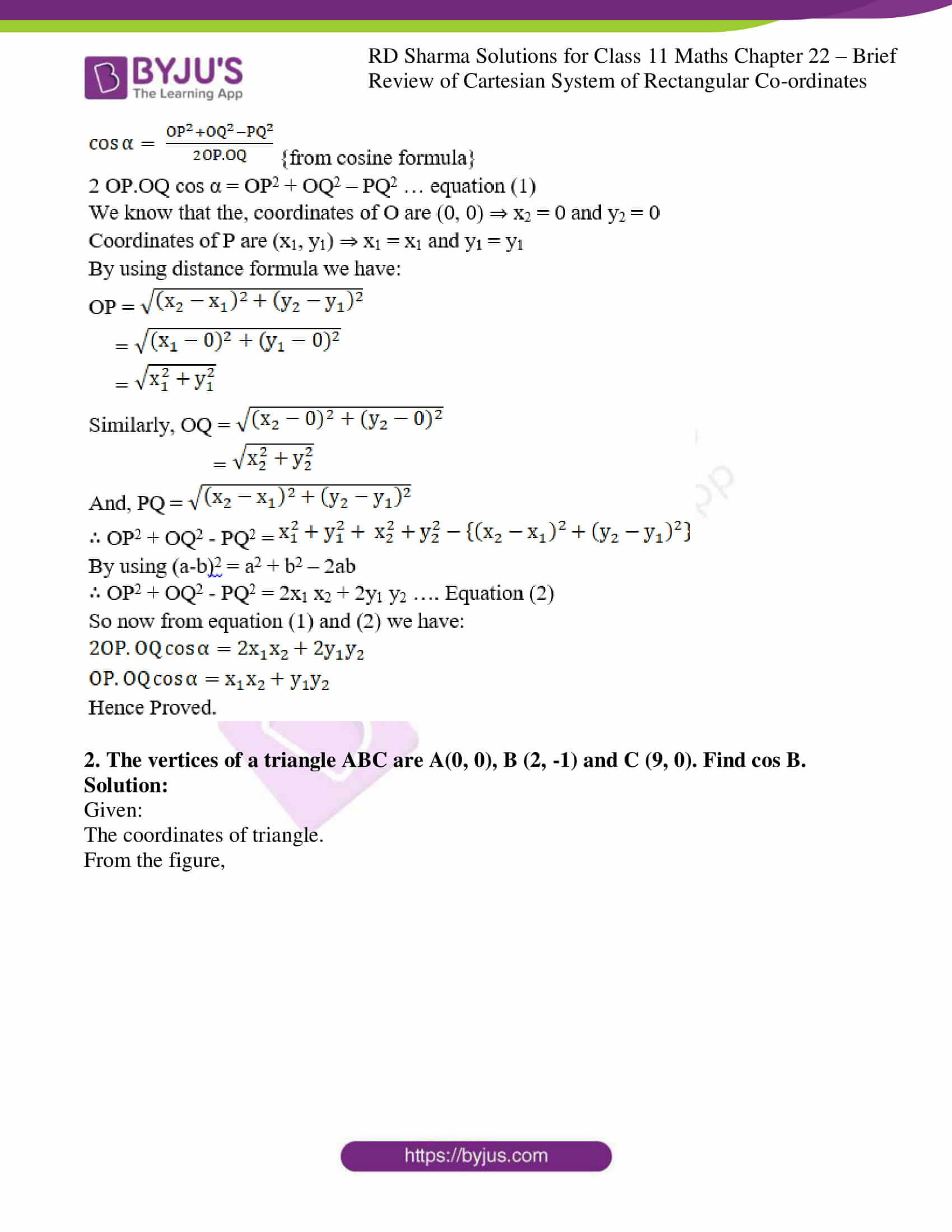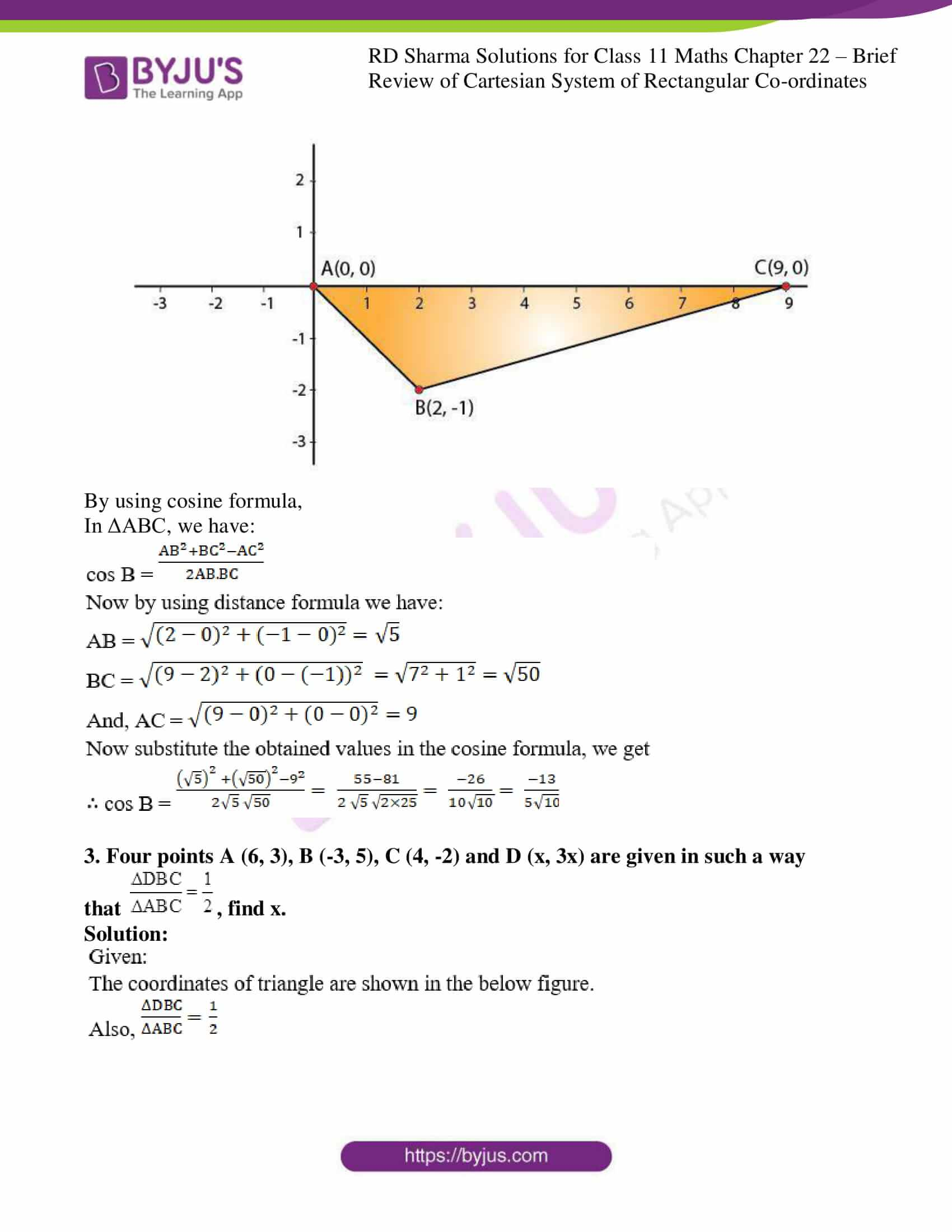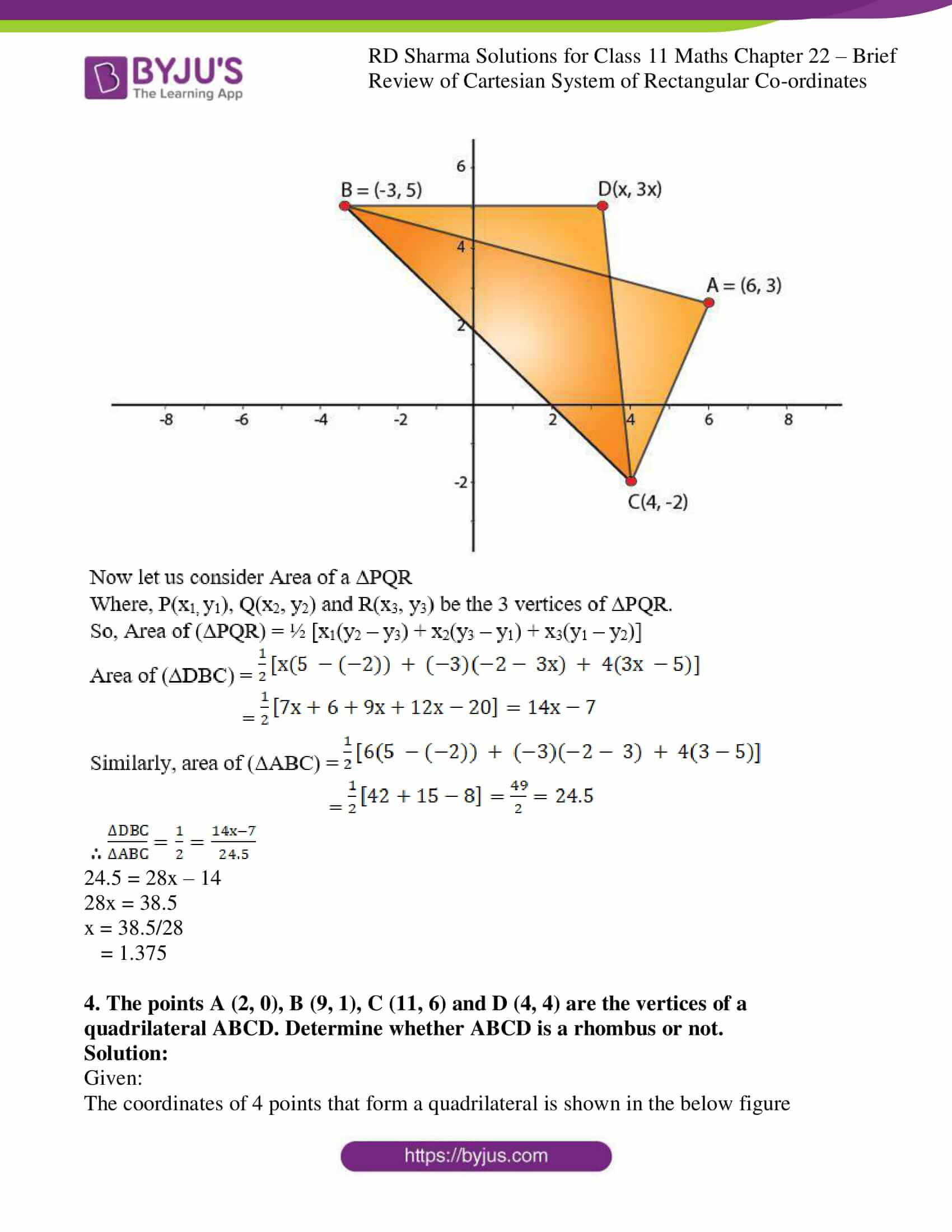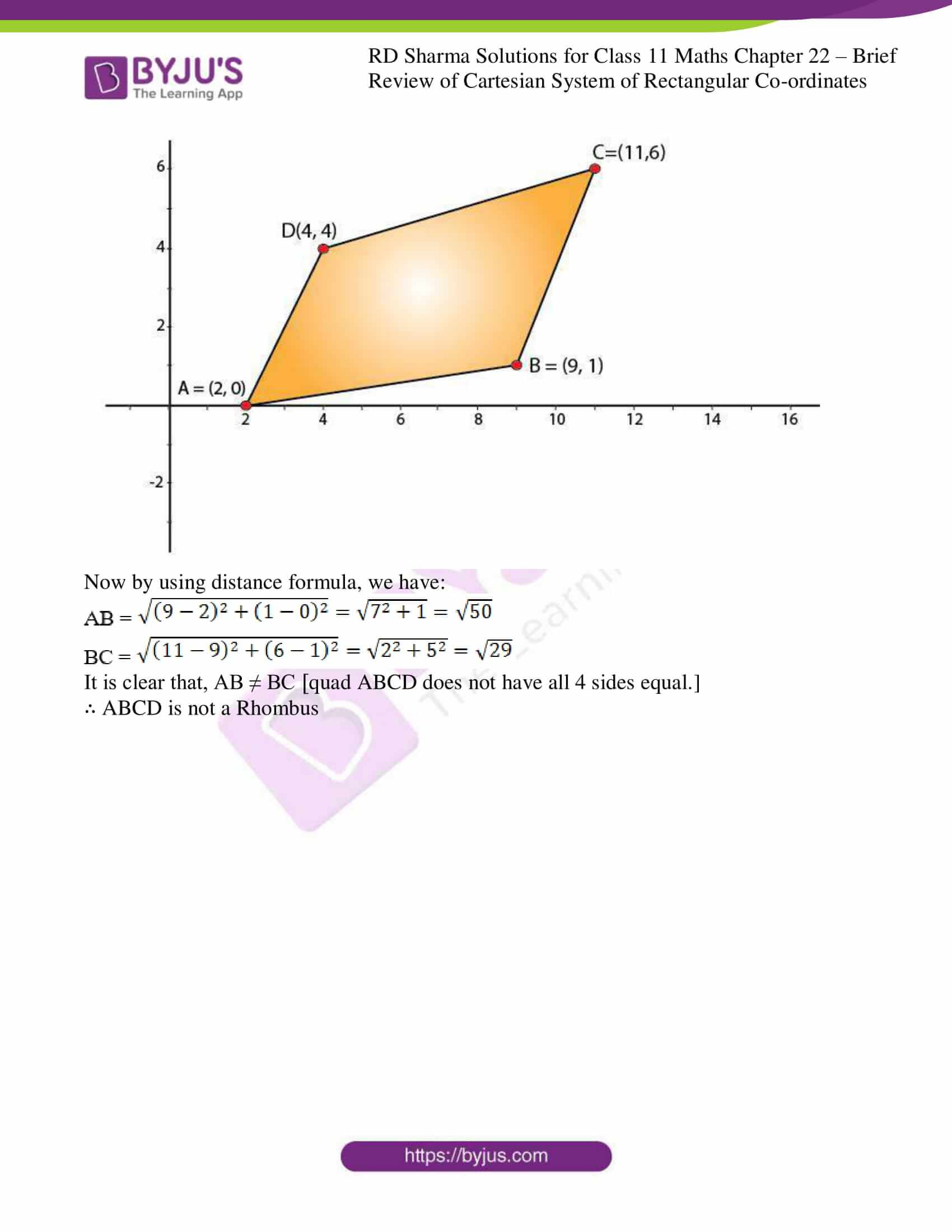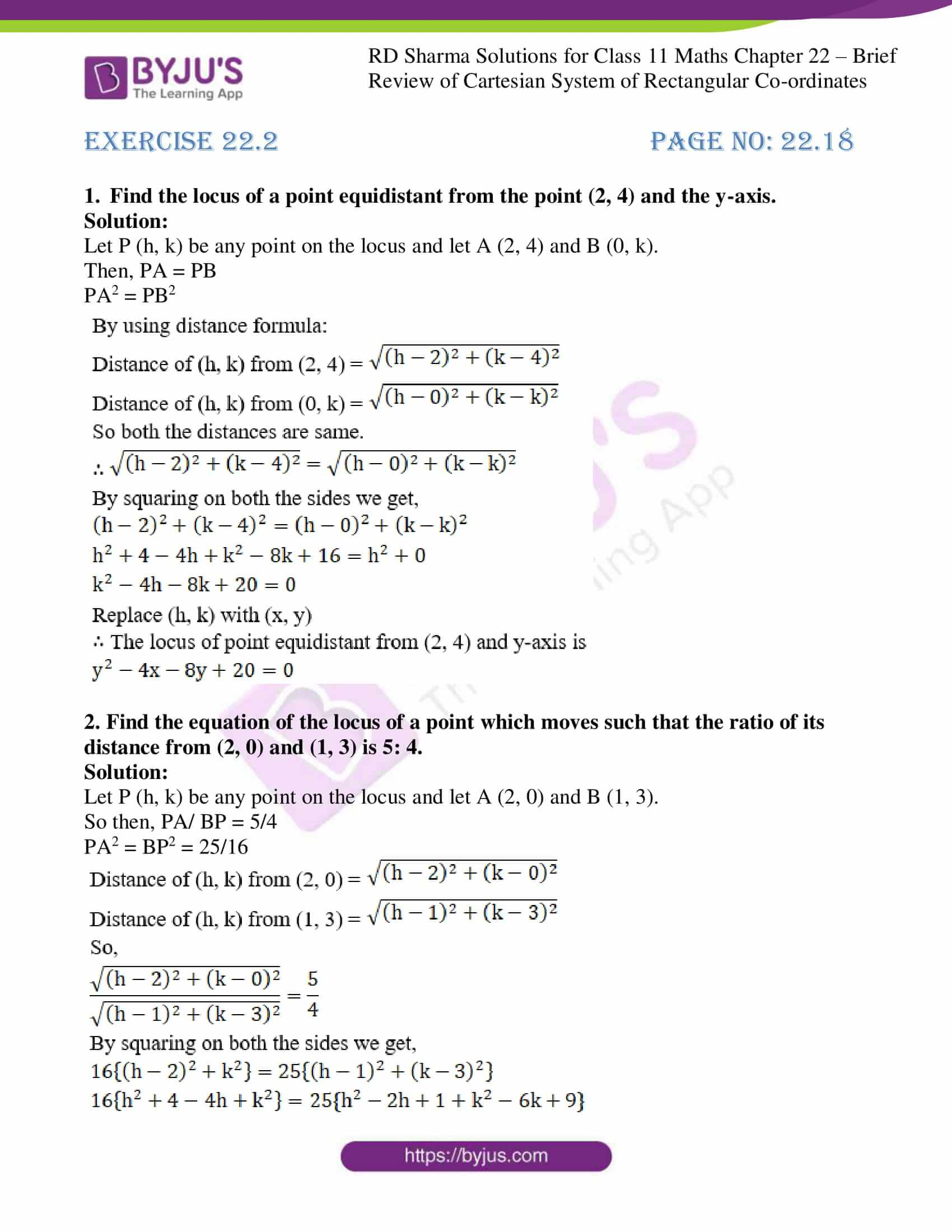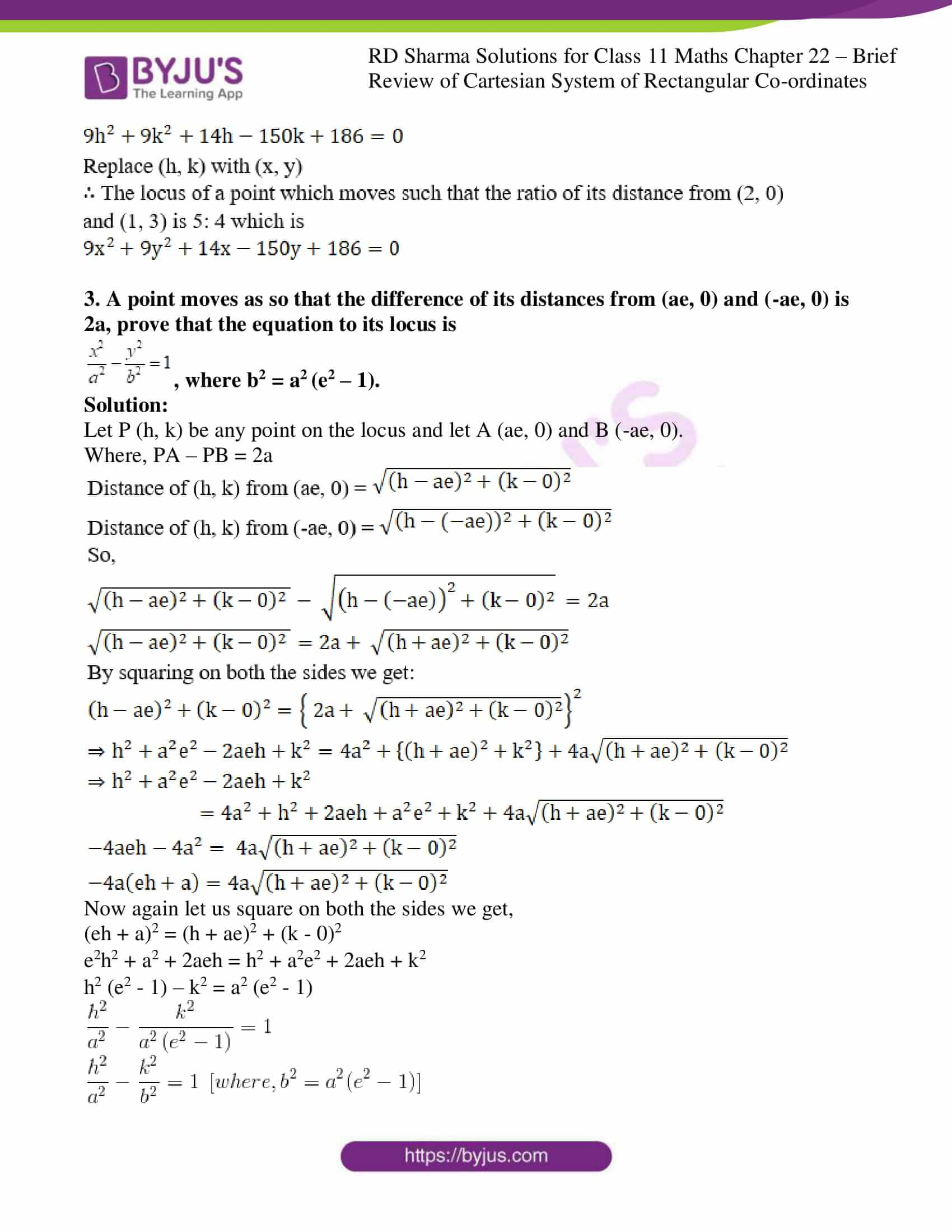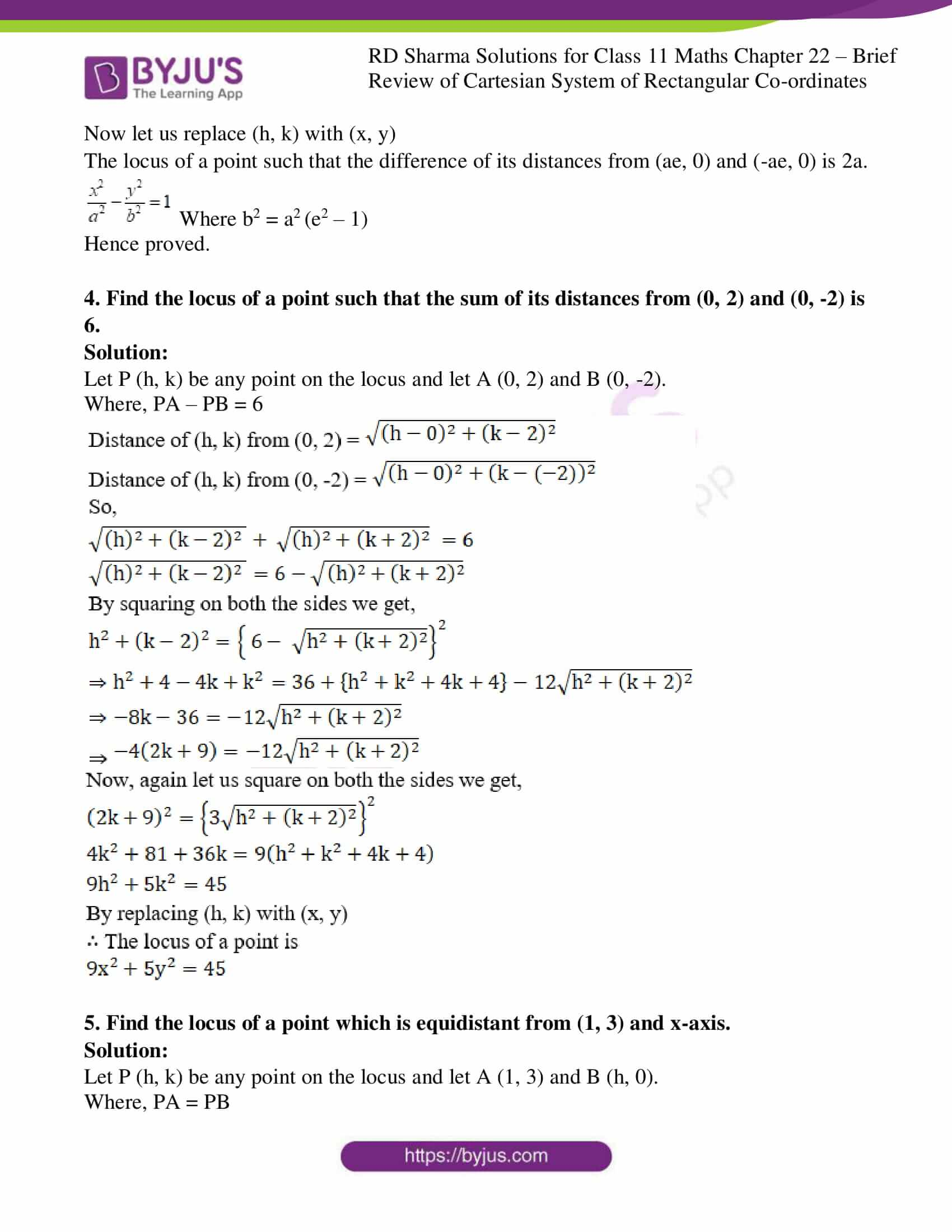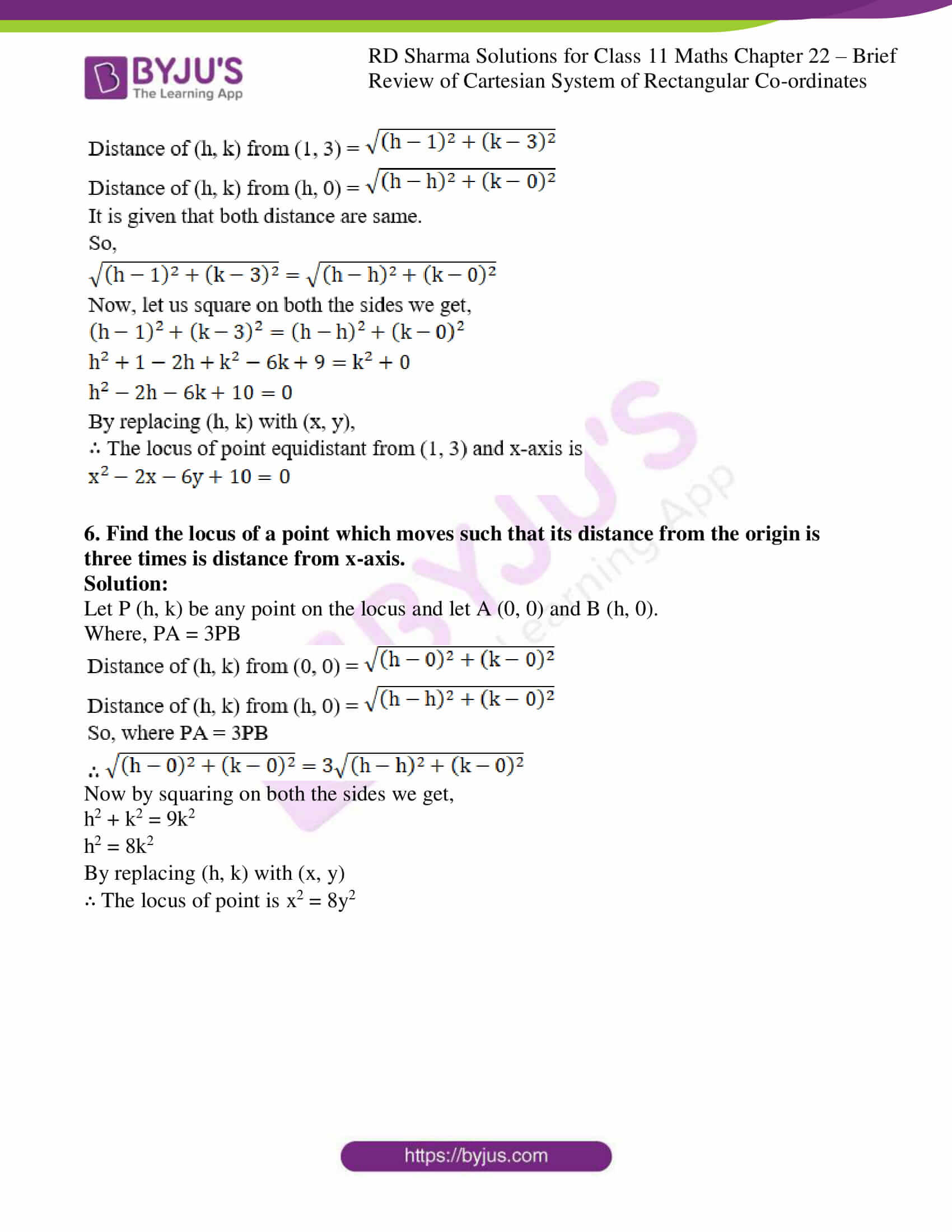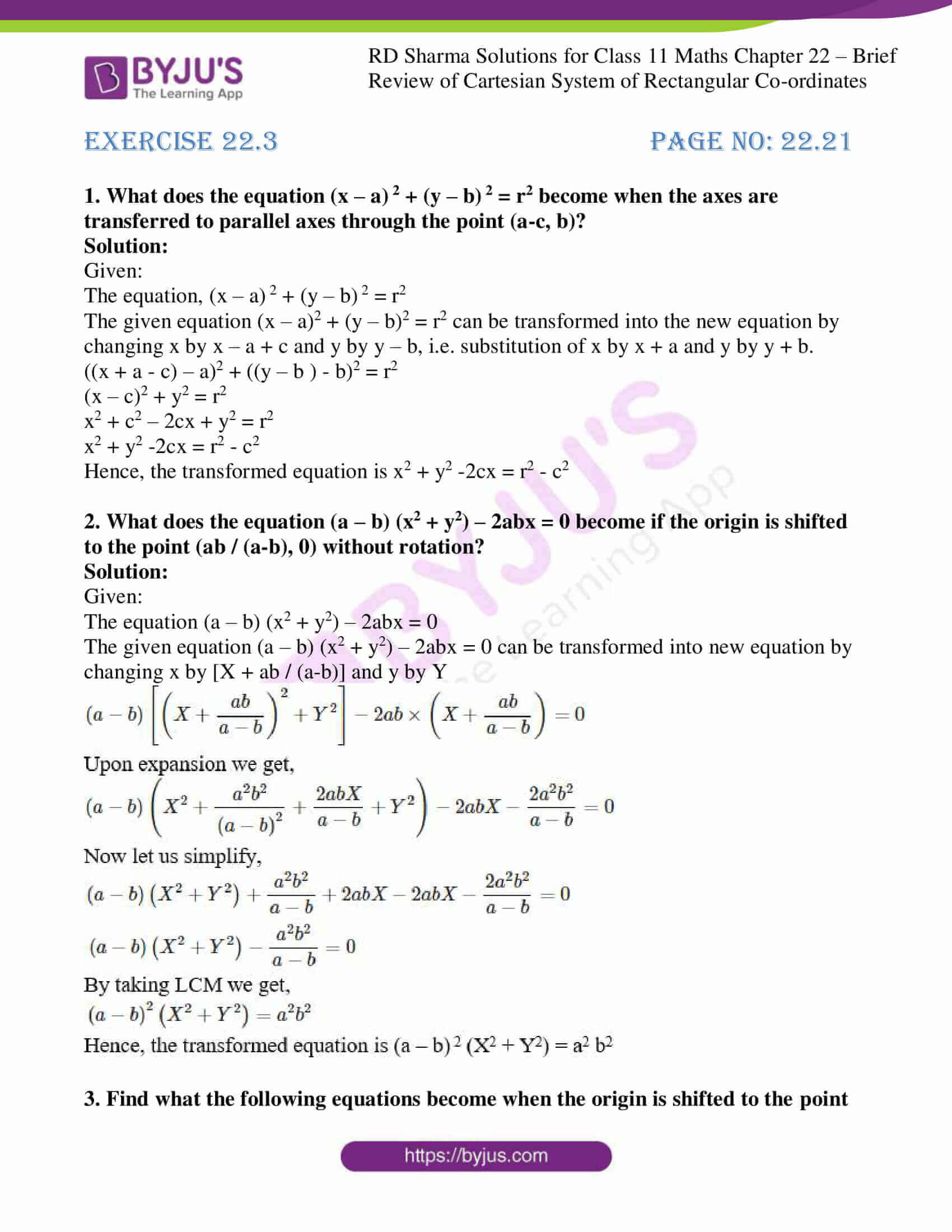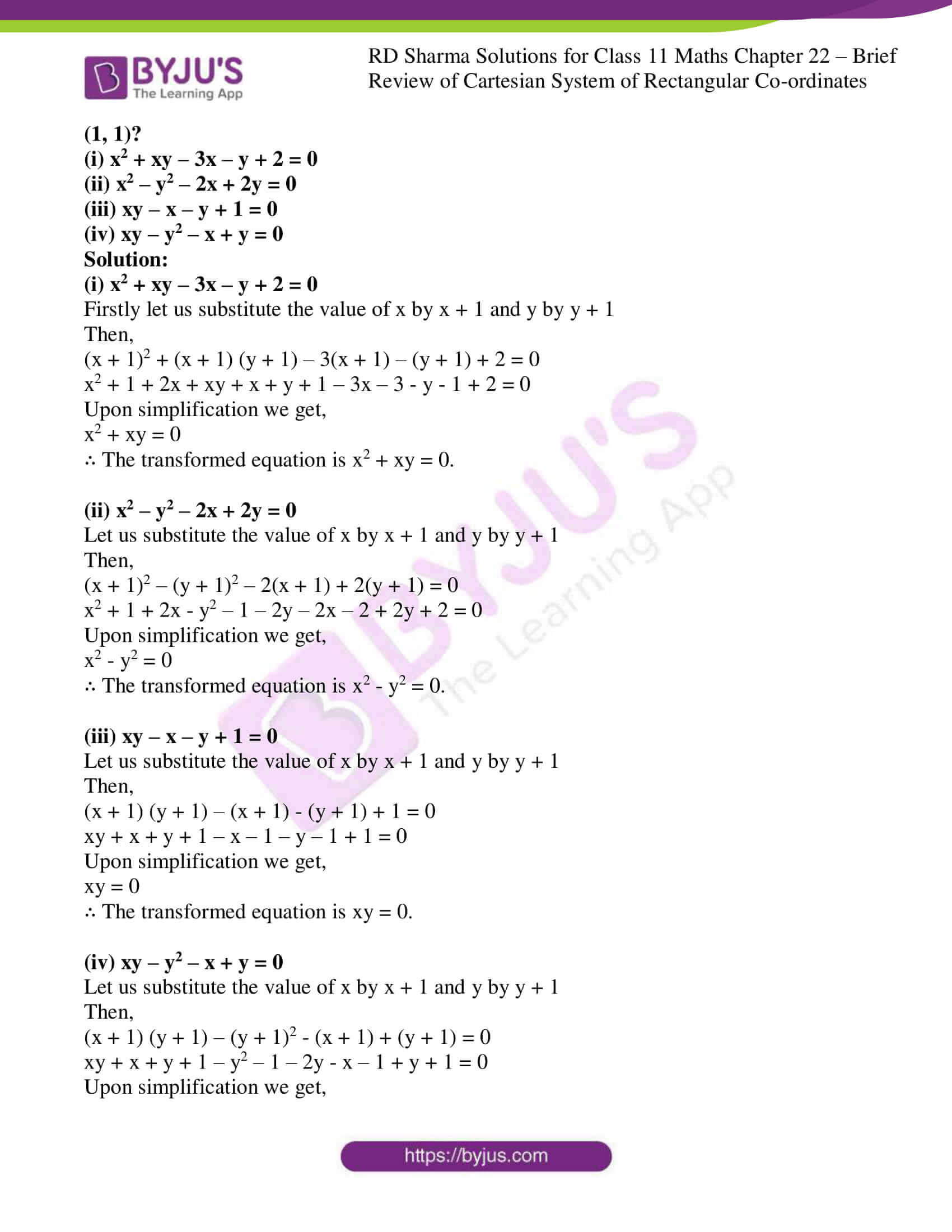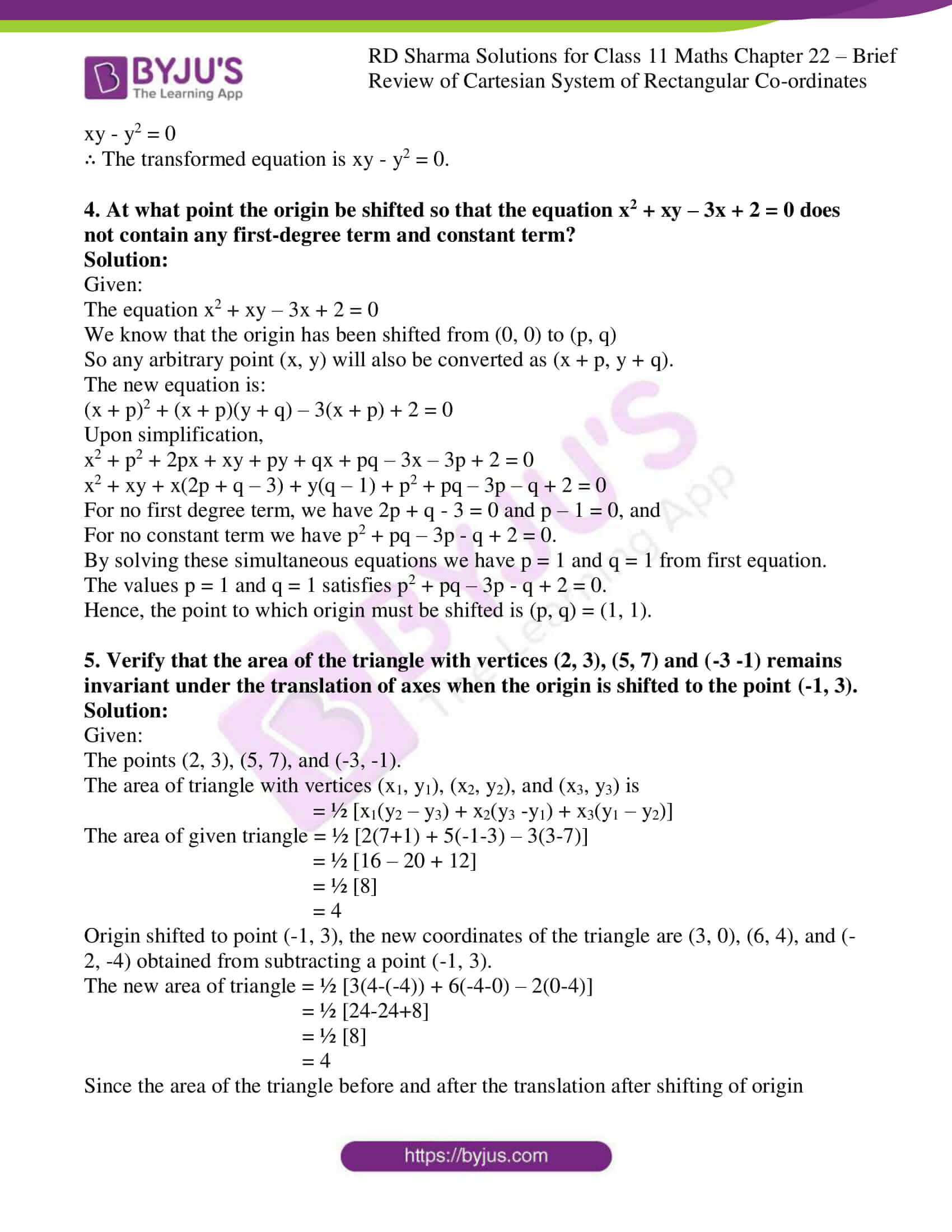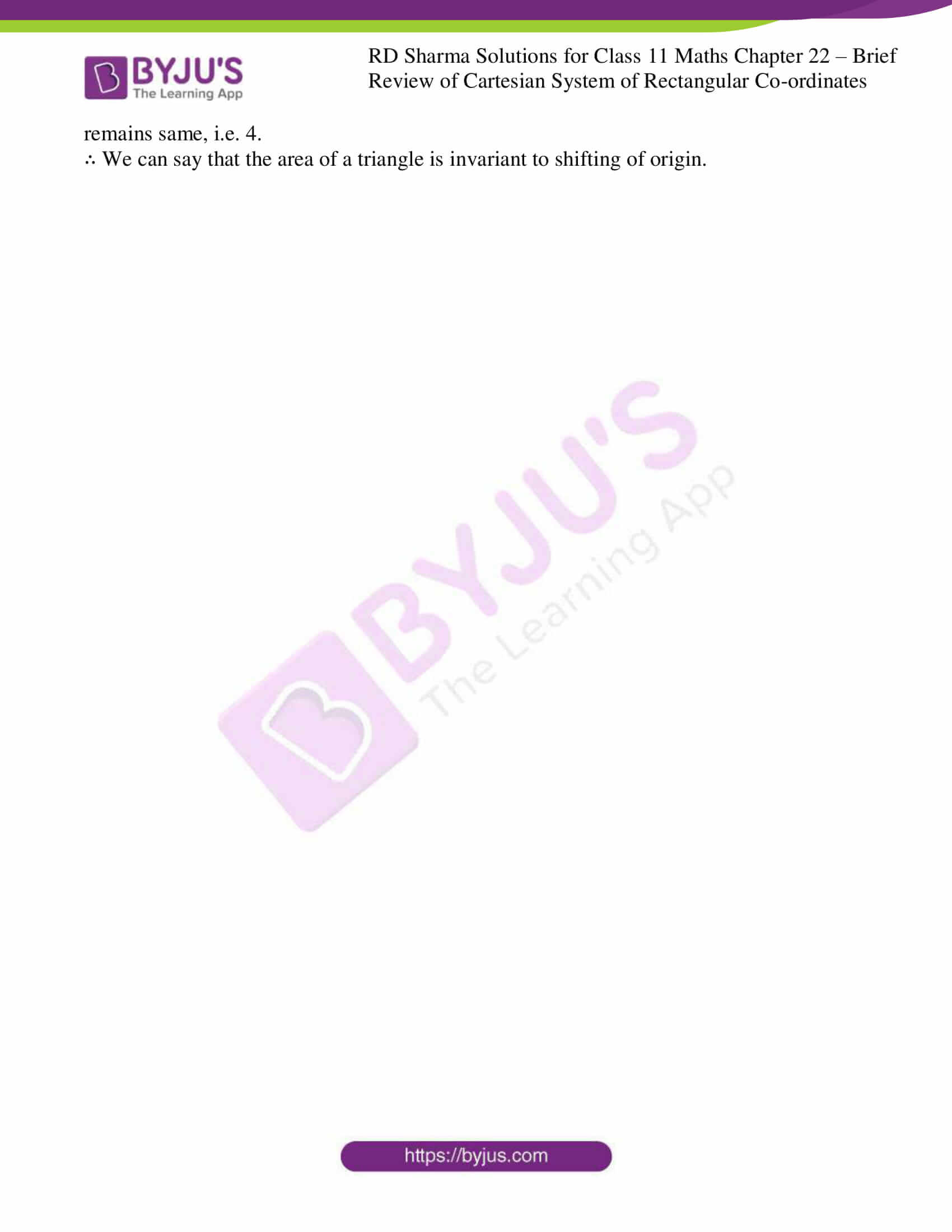### Access answers to RD Sharma Solutions for Class 11 Maths Chapter 22 – Brief review of Cartesian System of Rectangular Coordinates

EXERCISE 22.1 PAGE NO: 22.12

1. If the line segment joining the points P(x1, y1) and Q(x2, y2) subtends an angle α at the origin O, prove that : OP. OQ cos α = x1 x2 + y1 y2.

Solution:

Given,

Two points P and Q subtends an angle α at the origin as shown in figure: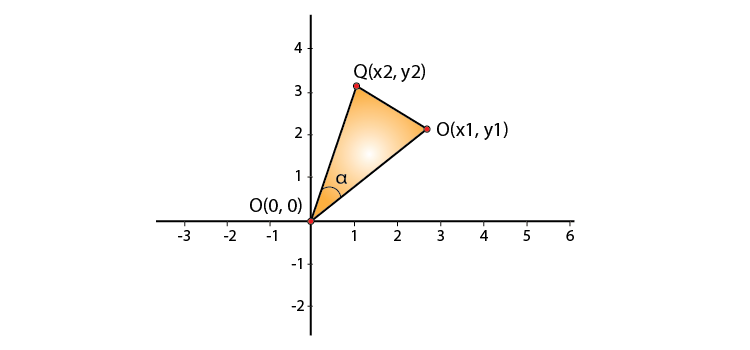From figure we can see that points O, P and Q forms a triangle.

Clearly in ΔOPQ we have: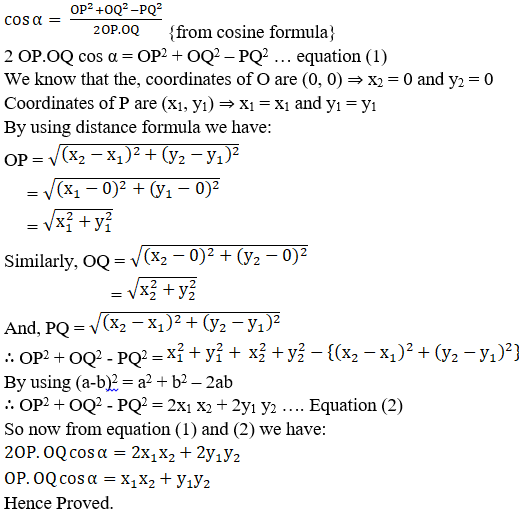2. The vertices of a triangle ABC are A(0, 0), B (2, -1) and C (9, 0). Find cos B.

Solution:

Given:

The coordinates of triangle.

From the figure,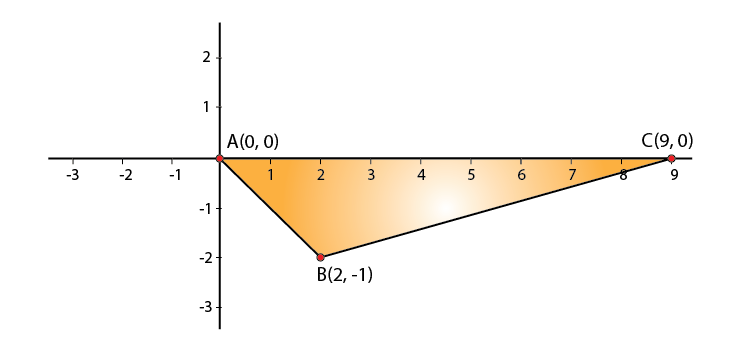By using cosine formula,

In ΔABC, we have: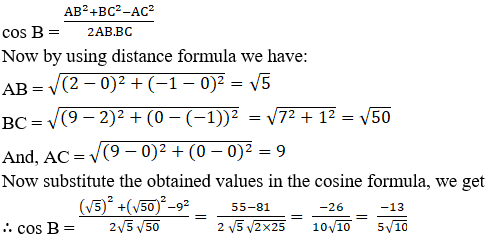3. Four points A (6, 3), B (-3, 5), C (4, -2) and D (x, 3x) are given in such a way that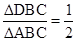, find x.

Solution: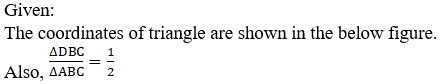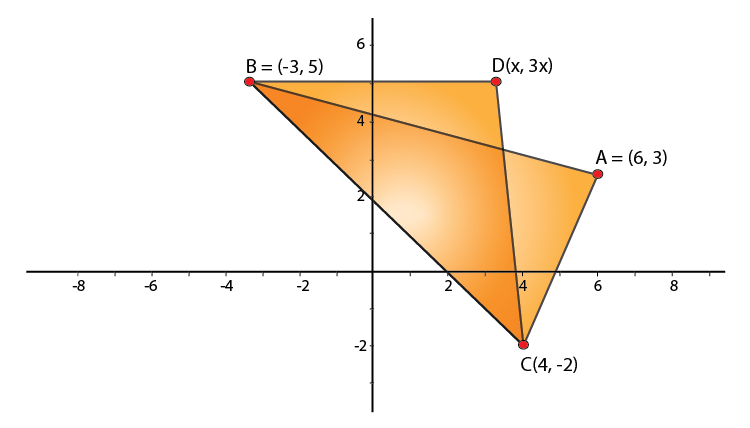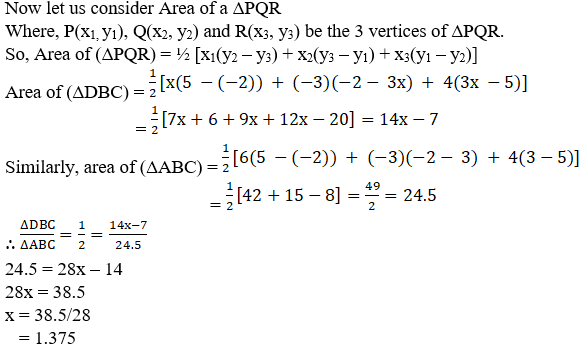24.5 = 28x – 14

28x = 38.5

x = 38.5/28

= 1.375

4. The points A (2, 0), B (9, 1), C (11, 6) and D (4, 4) are the vertices of a quadrilateral ABCD. Determine whether ABCD is a rhombus or not.

Solution:

Given:

The coordinates of 4 points that form a quadrilateral is shown in the below figure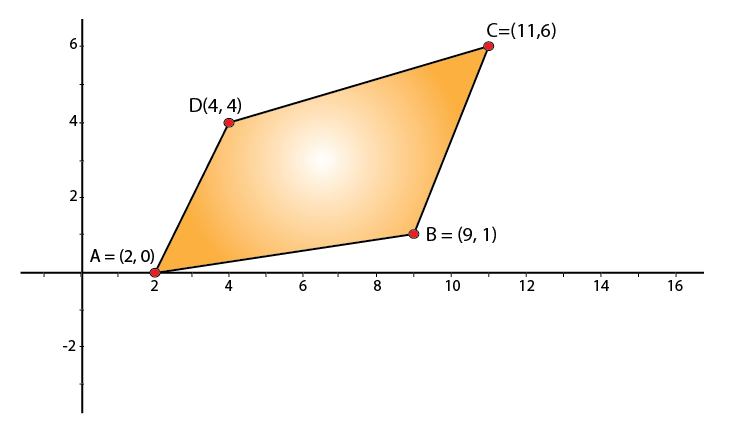Now by using distance formula, we have: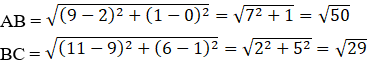It is clear that, AB ≠ BC [quad ABCD does not have all 4 sides equal.]

∴ ABCD is not a Rhombus

EXERCISE 22.2 PAGE NO: 22.18

1. Find the locus of a point equidistant from the point (2, 4) and the y-axis.

Solution:

Let P (h, k) be any point on the locus and let A (2, 4) and B (0, k).

Then, PA = PB

PA2 = PB2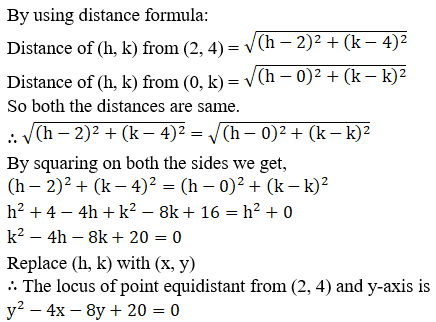2. Find the equation of the locus of a point which moves such that the ratio of its distance from (2, 0) and (1, 3) is 5: 4.

Solution:

Let P (h, k) be any point on the locus and let A (2, 0) and B (1, 3).

So then, PA/ BP = 5/4

PA2 = BP2 = 25/16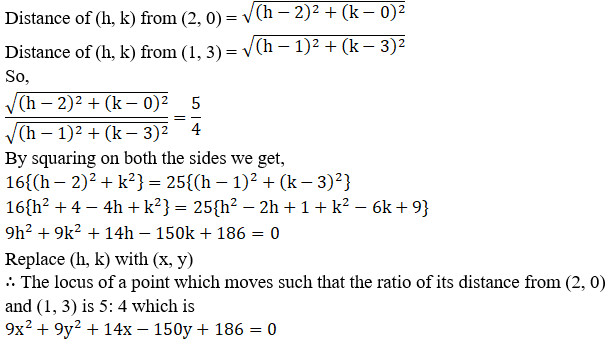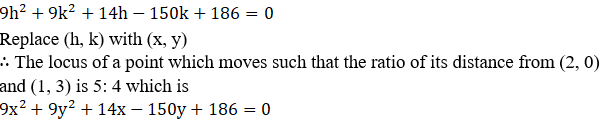3. A point moves as so that the difference of its distances from (ae, 0) and (-ae, 0) is 2a, prove that the equation to its locus is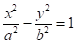, where b2 = a2 (e2 – 1).

Solution:

Let P (h, k) be any point on the locus and let A (ae, 0) and B (-ae, 0).

Where, PA – PB = 2a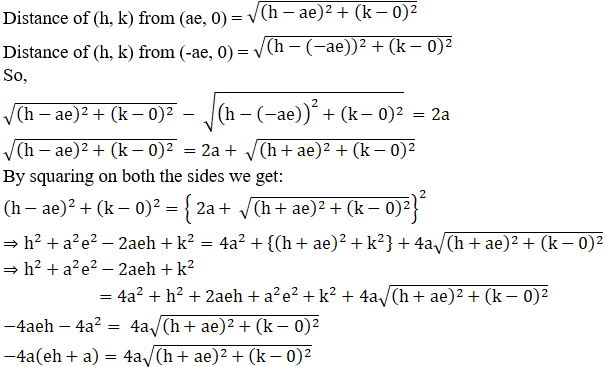Now again let us square on both the sides we get,

(eh + a)2 = (h + ae)2 + (k – 0)2

e2h2 + a2 + 2aeh = h2 + a2e2 + 2aeh + k2

h2 (e2 – 1) – k2 = a2 (e2 – 1)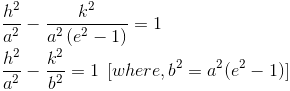Now let us replace (h, k) with (x, y)

The locus of a point such that the difference of its distances from (ae, 0) and (-ae, 0) is 2a.Where b2 = a2 (e2 – 1)

Hence proved.

4. Find the locus of a point such that the sum of its distances from (0, 2) and (0, -2) is 6.

Solution:

Let P (h, k) be any point on the locus and let A (0, 2) and B (0, -2).

Where, PA – PB = 6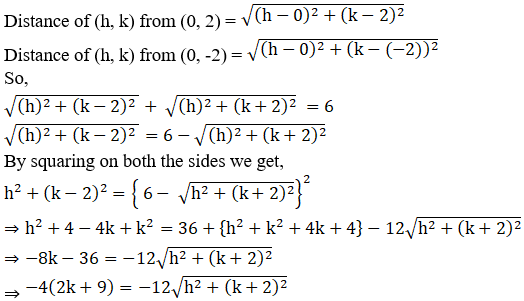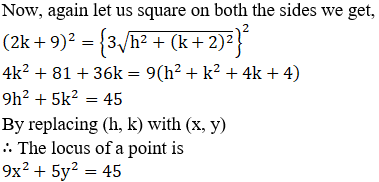5. Find the locus of a point which is equidistant from (1, 3) and x-axis.

Solution:

Let P (h, k) be any point on the locus and let A (1, 3) and B (h, 0).

Where, PA = PB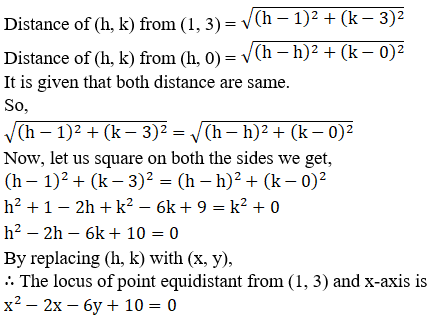6. Find the locus of a point which moves such that its distance from the origin is three times is distance from x-axis.

Solution:

Let P (h, k) be any point on the locus and let A (0, 0) and B (h, 0).

Where, PA = 3PB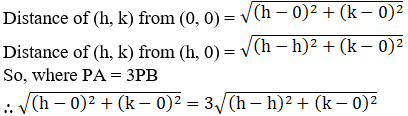Now by squaring on both the sides we get,

h2 + k2 = 9k2

h2 = 8k2

By replacing (h, k) with (x, y)

∴ The locus of point is x2 = 8y2

EXERCISE 22.3 PAGE NO: 22.21

1. What does the equation (x – a) 2 + (y – b) 2 = r2 become when the axes are transferred to parallel axes through the point (a-c, b)?

Solution:

Given:

The equation, (x – a) 2 + (y – b) 2 = r2

The given equation (x – a)2 + (y – b)2 = r2 can be transformed into the new equation by changing x by x – a + c and y by y – b, i.e. substitution of x by x + a and y by y + b.

((x + a – c) – a)2 + ((y – b ) – b)2 = r2

(x – c)2 + y2 = r2

x2 + c2 – 2cx + y2 = r2

x2 + y2 -2cx = r2 – c2

Hence, the transformed equation is x2 + y2 -2cx = r2 – c2

2. What does the equation (a – b) (x2 + y2) – 2abx = 0 become if the origin is shifted to the point (ab / (a-b), 0) without rotation?

Solution:

Given:

The equation (a – b) (x2 + y2) – 2abx = 0

The given equation (a – b) (x2 + y2) – 2abx = 0 can be transformed into new equation by changing x by [X + ab / (a-b)] and y by Y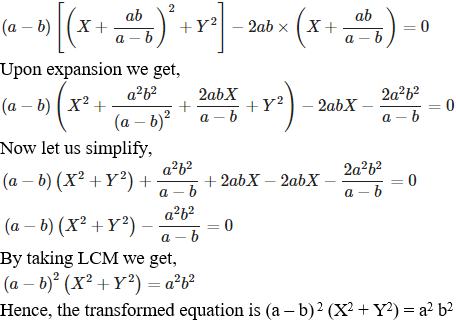3. Find what the following equations become when the origin is shifted to the point (1, 1)?
(i) x2 + xy – 3x – y + 2 = 0
(ii) x2 – y2 – 2x + 2y = 0
(iii) xy – x – y + 1 = 0
(iv) xy – y2 – x + y = 0

Solution:

(i) x2 + xy – 3x – y + 2 = 0

Firstly let us substitute the value of x by x + 1 and y by y + 1

Then,

(x + 1)2 + (x + 1) (y + 1) – 3(x + 1) – (y + 1) + 2 = 0

x2 + 1 + 2x + xy + x + y + 1 – 3x – 3 – y – 1 + 2 = 0

Upon simplification we get,

x2 + xy = 0

∴ The transformed equation is x2 + xy = 0.

(ii) x2 – y2 – 2x + 2y = 0

Let us substitute the value of x by x + 1 and y by y + 1

Then,

(x + 1)2 – (y + 1)2 – 2(x + 1) + 2(y + 1) = 0

x2 + 1 + 2x – y2 – 1 – 2y – 2x – 2 + 2y + 2 = 0

Upon simplification we get,

x2 – y2 = 0

∴ The transformed equation is x2 – y2 = 0.

(iii) xy – x – y + 1 = 0

Let us substitute the value of x by x + 1 and y by y + 1

Then,

(x + 1) (y + 1) – (x + 1) – (y + 1) + 1 = 0

xy + x + y + 1 – x – 1 – y – 1 + 1 = 0

Upon simplification we get,

xy = 0

∴ The transformed equation is xy = 0.

(iv) xy – y2 – x + y = 0

Let us substitute the value of x by x + 1 and y by y + 1

Then,

(x + 1) (y + 1) – (y + 1)2 – (x + 1) + (y + 1) = 0

xy + x + y + 1 – y2 – 1 – 2y – x – 1 + y + 1 = 0

Upon simplification we get,

xy – y2 = 0

∴ The transformed equation is xy – y2 = 0.

4. At what point the origin be shifted so that the equation x2 + xy – 3x + 2 = 0 does not contain any first-degree term and constant term?

Solution:

Given:

The equation x2 + xy – 3x + 2 = 0

We know that the origin has been shifted from (0, 0) to (p, q)

So any arbitrary point (x, y) will also be converted as (x + p, y + q).

The new equation is:

(x + p)2 + (x + p)(y + q) – 3(x + p) + 2 = 0

Upon simplification,

x2 + p2 + 2px + xy + py + qx + pq – 3x – 3p + 2 = 0

x2 + xy + x(2p + q – 3) + y(q – 1) + p2 + pq – 3p – q + 2 = 0

For no first degree term, we have 2p + q – 3 = 0 and p – 1 = 0, and

For no constant term we have p2 + pq – 3p – q + 2 = 0.

By solving these simultaneous equations we have p = 1 and q = 1 from first equation.

The values p = 1 and q = 1 satisfies p2 + pq – 3p – q + 2 = 0.

Hence, the point to which origin must be shifted is (p, q) = (1, 1).

5. Verify that the area of the triangle with vertices (2, 3), (5, 7) and (-3 -1) remains invariant under the translation of axes when the origin is shifted to the point (-1, 3).

Solution:

Given:

The points (2, 3), (5, 7), and (-3, -1).

The area of triangle with vertices (x1, y1), (x2, y2), and (x3, y3) is

= ½ [x1(y2 – y3) + x2(y3 -y1) + x3(y1 – y2)]

The area of given triangle = ½ [2(7+1) + 5(-1-3) – 3(3-7)]

= ½ [16 – 20 + 12]

= ½ 

= 4

Origin shifted to point (-1, 3), the new coordinates of the triangle are (3, 0), (6, 4), and (-2, -4) obtained from subtracting a point (-1, 3).

The new area of triangle = ½ [3(4-(-4)) + 6(-4-0) – 2(0-4)]

= ½ [24-24+8]

= ½ 

= 4

Since the area of the triangle before and after the translation after shifting of origin remains same, i.e. 4.

∴ We can say that the area of a triangle is invariant to shifting of origin.

### Also, access exercises of RD Sharma Solutions for Class 11 Maths Chapter 22 – Brief review of Cartesian System of Rectangular Coordinates

Exercise 22.1 Solutions

Exercise 22.2 Solutions

Exercise 22.3 Solutions

## Frequently Asked Questions on RD Sharma Solutions for Class 11 Maths Chapter 22

### Why should one choose the RD Sharma Solutions for Class 11 Maths Chapter 22 from BYJU’S?

We at BYJU’S provide 100% accurate answers so that the students can confidently score good marks in the board exams. Students who have doubts while answering the textbook questions can refer to the chapter solutions to get a clear idea about the overall concepts. The solutions are framed by the expert tutors after conducting a vast research on each concept. It mainly increases interest among students to learn new concepts without any difficulty.

### How should I prepare for the board exam using the RD Sharma Solutions for Class 11 Maths Chapter 22?

Students can learn the chapter and revise them on a regular basis to get a grip on the concepts. Students can refer to the syllabus and the correct study materials like the RD Sharma Solutions provided at BYJU’S should be chosen to score good marks in the exam. The syllabus for the academic year should be understood before the exam to know the marks weightage of each concept covered in the chapter. Students should refer to the solutions while learning a new chapter to understand the method of answering questions as per the latest CBSE guidelines.

### Does the BYJU’S RD Sharma Solutions for Class 11 Maths Chapter 22 provide accurate information for the board exam preparation?

Yes, the RD Sharma Solutions for Class 11 Maths Chapter 22 offered by BYJU’S is accurate enough based on the latest guidelines set by the CBSE board. The concepts are solved in an elaborate manner to help students understand them without any difficulty. The expert tutors have created the modules passionately so that students can learn new topics efficiently. Students can access the PDF format of syllabus as well as solutions which are available in BYJU’S website with a free download option and score well in the board exams.